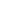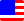Documents  >  Tutorial  >  group4
Operators of the 4th group

Purpose of this chapter.

Operators of the 4th group.

Operators of the 4th group are following.

 + x + y addition operator - x - y subtraction operator

These are arithmetic operators with low priority.

Addition operator is an operator that adds the right operand to the left operand. The operand must be an instance of the int, long, or real class.

The class of the result depends on the class of the operand.

If there is an instance of the real class in the operand, the class of the result is real.

For example,

real r0 = 123.5 + 10.0; // The left is real and the right is real. Therefore, result is real.

real r1 = 123.5 + 10; // The left is real and the right is int. Therefore, result is real.

real r2 = 123.5 + 10L; // The left is real and right is long. Therefore, result is real.

If there is no real class in the operand and there is an instance of the long class, the class of the result is long.

For example,

long l0 = 123L + 10L; // The left is long and the right is long. Therefore, result is long.

long l1 = 123L + 10; // The left is long and the right is int. Therefore, result is long.

Otherwise, the class of the result is int.

For example,

real i0 = 123 + 10; // The left is int and the right is int. Therefore, result is int.

If the value of the result exceeds the range of the class, an exception is thrown.

subtraction operator

Subtraction operator is an operator that subtracts the right operand from the left operand. The operand must be an instance of the int, long, or real class.

The class of the result depends on the class of the operand.

If there is an instance of the real class in the operand, the class of the result is real.

For example,

real r0 = 123.5 - 10.0; // The left is real and the right is real. Therefore, result is real.

real r1 = 123.5 - 10; // The left is real and the right is int. Therefore, result is real.

real r2 = 123.5 - 10L; // The left is real and right is long. Therefore, result is real.

If there is no real class in the operand and there is an instance of the long class, the class of the result is long.

For example,

long l0 = 123L - 10L; // The left is long and the right is long. Therefore, result is long.

long l1 = 123L - 10; // The left is long and the right is int. Therefore, result is long.

Otherwise, the class of the result is int.

For example,

real i0 = 123 - 10; // The left is int and the right is int. Therefore, result is int.

If the value of the result exceeds the range of the class, an exception is thrown.

concatenation operator

If the left and right operands of the + symbol are string class, The + symbol is a concatenation operator, not an addition operator.

The concatenation operator returns an new instance of the string class that concatenated the right operand to the left operand.

For example,

string s = "Hellow " + "world!"; // The result is "Hellow world!".